Precalculus

# Chapter 8

PrecalculusChapter 8

### 8.1Non-right Triangles: Law of Sines

1.

$α= 98 ∘ a=34.6 β= 39 ∘ b=22 γ= 43 ∘ c=23.8 α= 98 ∘ a=34.6 β= 39 ∘ b=22 γ= 43 ∘ c=23.8$

2.

Solution 1

$α=80° a=120 β≈83.2° b=121 γ≈16.8° c≈35.2 α=80° a=120 β≈83.2° b=121 γ≈16.8° c≈35.2$

Solution 2

$α ′ =80° a ′ =120 β ′ ≈96.8° b ′ =121 γ ′ ≈3.2° c ′ ≈6.8 α ′ =80° a ′ =120 β ′ ≈96.8° b ′ =121 γ ′ ≈3.2° c ′ ≈6.8$
3.

$β≈5.7°,γ≈94.3°,c≈101.3 β≈5.7°,γ≈94.3°,c≈101.3$

4.

two

5.

about $8.2 8.2$ square feet

6.

161.9 yd.

### 8.2Non-right Triangles: Law of Cosines

1.

$a≈14.9, a≈14.9,$ $β≈23.8°,β≈23.8°,$ $γ≈126.2°. γ≈126.2°.$

2.

$α≈27.7°, α≈27.7°,$ $β≈40.5°,β≈40.5°,$ $γ≈111.8° γ≈111.8°$

3.

Area = 552 square feet

4.

### 8.3Polar Coordinates

1.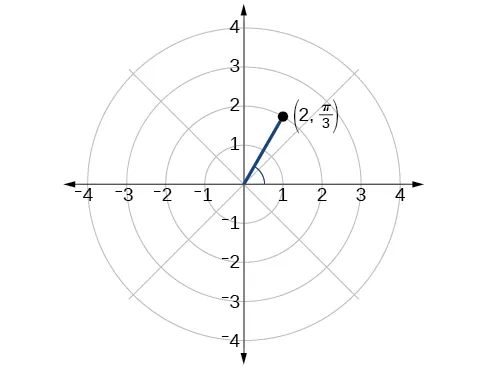2.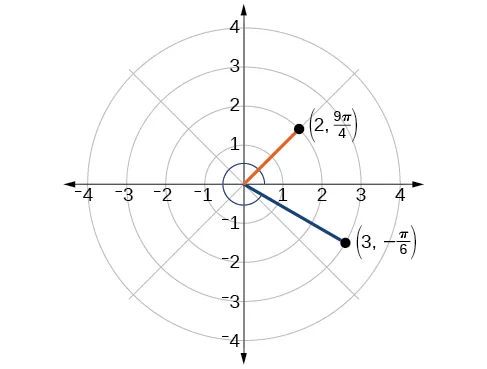3.

$( x,y )=( 1 2 ,− 3 2 ) ( x,y )=( 1 2 ,− 3 2 )$

4.

$r= 3 r= 3$

5.

$x 2 + y 2 =2y x 2 + y 2 =2y$ or, in the standard form for a circle, $x 2 + ( y−1 ) 2 =1 x 2 + ( y−1 ) 2 =1$

### 8.4Polar Coordinates: Graphs

1.

The equation fails the symmetry test with respect to the line $θ= π 2 θ= π 2$ and with respect to the pole. It passes the polar axis symmetry test.

2.

Tests will reveal symmetry about the polar axis. The zero is $( 0, π 2 ), ( 0, π 2 ),$ and the maximum value is $(3,0). (3,0).$

3.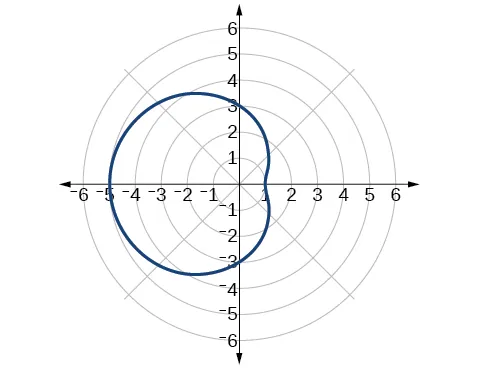4.

The graph is a rose curve, $n n$ even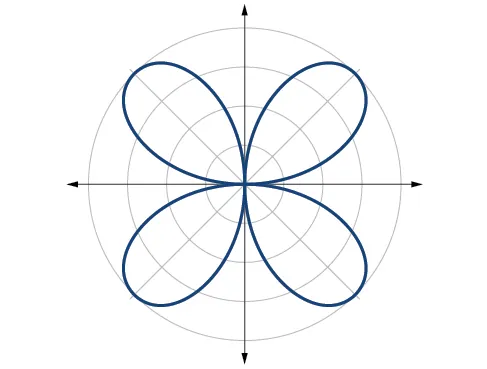5.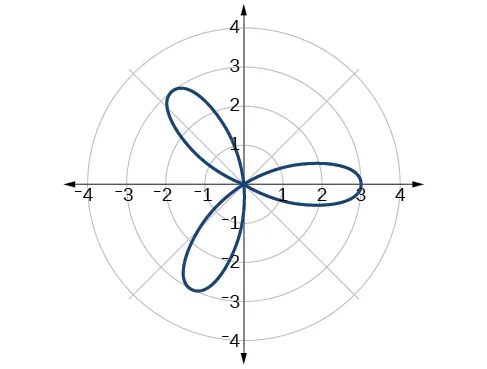Rose curve, $n n$ odd

6.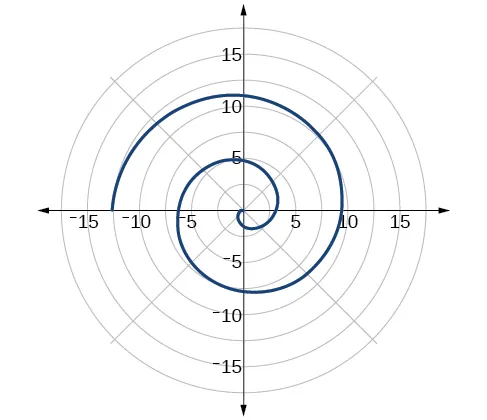### 8.5Polar Form of Complex Numbers

1.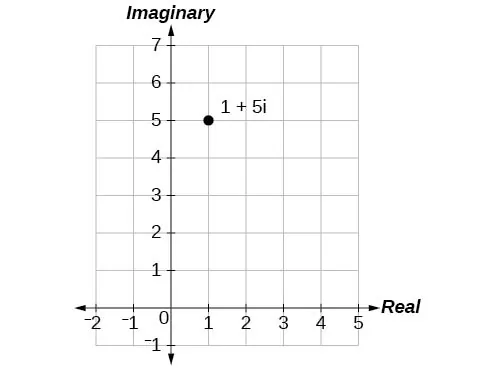2.

13

3.

$| z |= 50 =5 2 | z |= 50 =5 2$

4.

$z=3( cos( π 2 )+isin( π 2 ) ) z=3( cos( π 2 )+isin( π 2 ) )$

5.

$z=2( cos( π 6 )+isin( π 6 ) ) z=2( cos( π 6 )+isin( π 6 ) )$

6.

$z=2 3 −2i z=2 3 −2i$

7.

$z 1 z 2 =−4 3 ; z 1 z 2 =− 3 2 + 3 2 i z 1 z 2 =−4 3 ; z 1 z 2 =− 3 2 + 3 2 i$

8.

$z 0 =2(cos(30°)+isin(30°)) z 0 =2(cos(30°)+isin(30°))$

$z 1 =2(cos(120°)+isin(120°)) z 1 =2(cos(120°)+isin(120°))$

$z 2 =2(cos(210°)+isin(210°)) z 2 =2(cos(210°)+isin(210°))$

$z 3 =2(cos(300°)+isin(300°)) z 3 =2(cos(300°)+isin(300°))$

### 8.6Parametric Equations

1.
 $t t$ $x( t ) x( t )$ $y( t ) y( t )$ $−1 −1$ $−4 −4$ $2 2$ $0 0$ $−3 −3$ $4 4$ $1 1$ $−2 −2$ $6 6$ $2 2$ $−1 −1$ $8 8$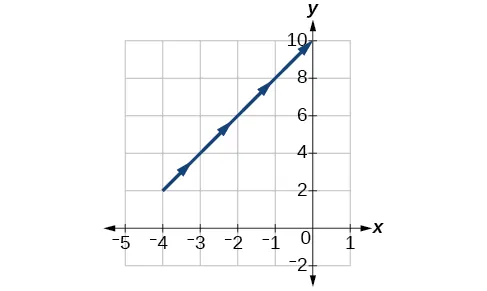2.

$x(t)= t 3 −2t y(t)=t x(t)= t 3 −2t y(t)=t$

3.

$y=5− 1 2 x−3 y=5− 1 2 x−3$

4.

$y=ln x y=ln x$

5.

$x 2 4 + y 2 9 =1 x 2 4 + y 2 9 =1$

6.

$y= x 2 y= x 2$

### 8.7Parametric Equations: Graphs

1.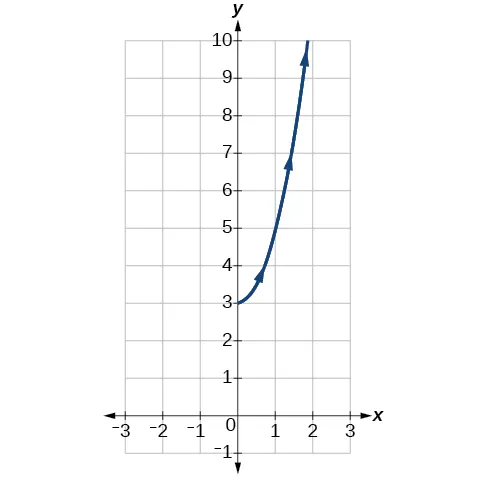2.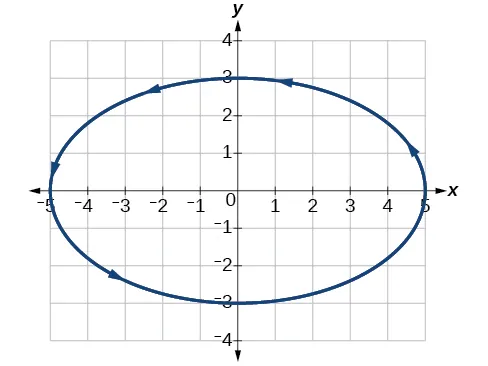3.

The graph of the parametric equations is in red and the graph of the rectangular equation is drawn in blue dots on top of the parametric equations.### 8.8Vectors

1.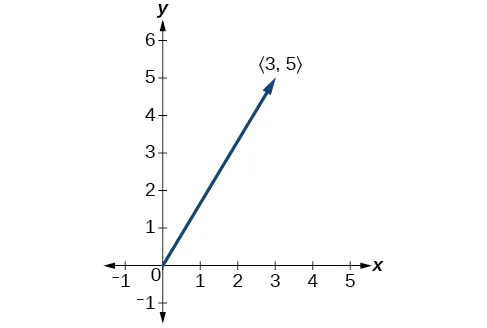2.

$3u=〈 15,12 〉 3u=〈 15,12 〉$

3.

$u=8i−11j u=8i−11j$

4.

$v= 34 cos(59°)i+ 34 sin(59°)j v= 34 cos(59°)i+ 34 sin(59°)j$

Magnitude = $34 34$

$θ= tan −1 ( 5 3 )=59.04° θ= tan −1 ( 5 3 )=59.04°$

### 8.1 Section Exercises

1.

The altitude extends from any vertex to the opposite side or to the line containing the opposite side at a 90° angle.

3.

When the known values are the side opposite the missing angle and another side and its opposite angle.

5.

A triangle with two given sides and a non-included angle.

7.

$β=72°,a≈12.0,b≈19.9 β=72°,a≈12.0,b≈19.9$

9.

$γ=20°,b≈4.5,c≈1.6 γ=20°,b≈4.5,c≈1.6$

11.

$b≈3.78 b≈3.78$

13.

$c≈13.70 c≈13.70$

15.

one triangle, $α≈50.3°,β≈16.7°,a≈26.7 α≈50.3°,β≈16.7°,a≈26.7$

17.

two triangles, $γ≈54.3°,β≈90.7°,b≈20.9 γ≈54.3°,β≈90.7°,b≈20.9$ or $γ ′ ≈125.7°, β ′ ≈19.3°, b ′ ≈6.9 γ ′ ≈125.7°, β ′ ≈19.3°, b ′ ≈6.9$

19.

two triangles, $β≈75.7°,γ≈61.3°,b≈9.9 β≈75.7°,γ≈61.3°,b≈9.9$ or $β ′ ≈18.3°, γ ′ ≈118.7°, b ′ ≈3.2 β ′ ≈18.3°, γ ′ ≈118.7°, b ′ ≈3.2$

21.

two triangles, $α≈143.2°,β≈26.8°,a≈17.3 α≈143.2°,β≈26.8°,a≈17.3$ or $α ′ ≈16.8°, β ′ ≈153.2°, a ′ ≈8.3 α ′ ≈16.8°, β ′ ≈153.2°, a ′ ≈8.3$

23.

no triangle possible

25.

$A≈47.8° A≈47.8°$ or $A ′ ≈ 132.2° A ′ ≈ 132.2°$

27.

$8.6 8.6$

29.

$370.9 370.9$

31.

$12.3 12.3$

33.

$12.2 12.2$

35.

$16.0 16.0$

37.

$29.7° 29.7°$

39.

$x=76.9°orx=103.1° x=76.9°orx=103.1°$

41.

$110.6° 110.6°$

43.

$A≈39.4,C≈47.6,BC≈20.7 A≈39.4,C≈47.6,BC≈20.7$

45.

$57.1 57.1$

47.

$42.0 42.0$

49.

$430.2 430.2$

51.

$10.1 10.1$

53.

$AD≈13.8 AD≈13.8$

55.

$AB≈2.8 AB≈2.8$

57.

$L≈49.7,N≈56.3,LN≈5.8 L≈49.7,N≈56.3,LN≈5.8$

59.

51.4 feet

61.

The distance from the satellite to station $A A$ is approximately 1716 miles. The satellite is approximately 1706 miles above the ground.

63.

2.6 ft

65.

5.6 km

67.

371 ft

69.

5936 ft

71.

24.1 ft

73.

19,056 ft2

75.

445,624 square miles

77.

8.65 ft2

### 8.2 Section Exercises

1.

two sides and the angle opposite the missing side.

3.

$s s$ is the semi-perimeter, which is half the perimeter of the triangle.

5.

The Law of Cosines must be used for any oblique (non-right) triangle.

7.

11.3

9.

34.7

11.

26.7

13.

257.4

15.

not possible

17.

95.5°

19.

26.9°

21.

$B≈45.9°,C≈99.1°,a≈6.4 B≈45.9°,C≈99.1°,a≈6.4$

23.

$A≈20.6°,B≈38.4°,c≈51.1 A≈20.6°,B≈38.4°,c≈51.1$

25.

$A≈37.8°,B≈43.8,C≈98.4° A≈37.8°,B≈43.8,C≈98.4°$

27.

177.56 in2

29.

0.04 m2

31.

0.91 yd2

33.

3.0

35.

29.1

37.

0.5

39.

70.7°

41.

77.4°

43.

25.0

45.

9.3

47.

43.52

49.

1.41

51.

0.14

53.

18.3

55.

48.98

57.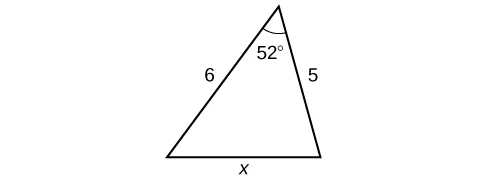59.

7.62

61.

85.1

63.

24.0 km

65.

99.9 ft

67.

37.3 miles

69.

2371 miles

71.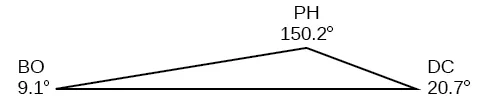73.

292.4 miles

75.

65.4 cm2

77.

468 ft2

### 8.3 Section Exercises

1.

For polar coordinates, the point in the plane depends on the angle from the positive x-axis and distance from the origin, while in Cartesian coordinates, the point represents the horizontal and vertical distances from the origin. For each point in the coordinate plane, there is one representation, but for each point in the polar plane, there are infinite representations.

3.

Determine $θ θ$ for the point, then move $r r$ units from the pole to plot the point. If $r r$ is negative, move $r r$ units from the pole in the opposite direction but along the same angle. The point is a distance of $r r$ away from the origin at an angle of $θ θ$ from the polar axis.

5.

The point $( −3, π 2 ) ( −3, π 2 )$ has a positive angle but a negative radius and is plotted by moving to an angle of $π 2 π 2$ and then moving 3 units in the negative direction. This places the point 3 units down the negative y-axis. The point $( 3,− π 2 ) ( 3,− π 2 )$ has a negative angle and a positive radius and is plotted by first moving to an angle of $− π 2 − π 2$ and then moving 3 units down, which is the positive direction for a negative angle. The point is also 3 units down the negative y-axis.

7.

$( −5,0 ) ( −5,0 )$

9.

$( − 3 3 2 ,− 3 2 ) ( − 3 3 2 ,− 3 2 )$

11.

$( 2 5 ,0.464 ) ( 2 5 ,0.464 )$

13.

$( 34 ,5.253 ) ( 34 ,5.253 )$

15.

$( 8 2 , π 4 ) ( 8 2 , π 4 )$

17.

$r=4cscθ r=4cscθ$

19.

$r= sinθ 2co s 4 θ 3 r= sinθ 2co s 4 θ 3$

21.

$r=3cosθ r=3cosθ$

23.

$r= 3sinθ cos( 2θ ) r= 3sinθ cos( 2θ )$

25.

$r= 9sinθ cos 2 θ r= 9sinθ cos 2 θ$

27.

$r= 1 9cosθsinθ r= 1 9cosθsinθ$

29.

$x 2 + y 2 =4x x 2 + y 2 =4x$ or $( x−2 ) 2 4 + y 2 4 =1; ( x−2 ) 2 4 + y 2 4 =1;$ circle

31.

$3y+x=6; 3y+x=6;$ line

33.

$y=3; y=3;$ line

35.

$xy=4; xy=4;$ hyperbola

37.

$x 2 + y 2 =4; x 2 + y 2 =4;$ circle

39.

$x−5y=3; x−5y=3;$ line

41.

$( 3, 3π 4 ) ( 3, 3π 4 )$

43.

$( 5,π ) ( 5,π )$

45.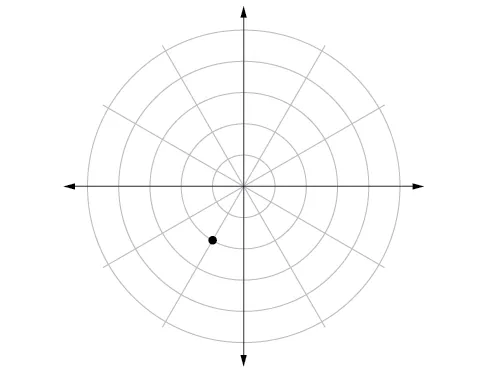47.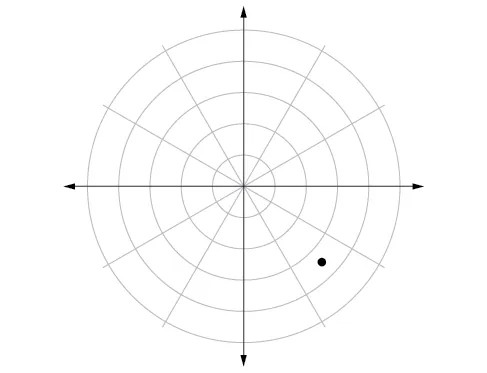49.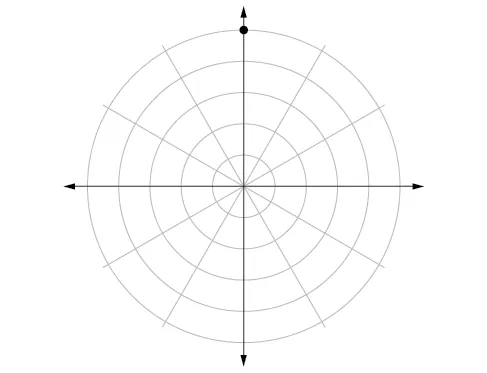51.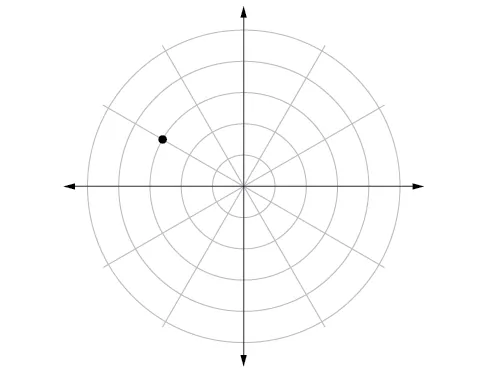53.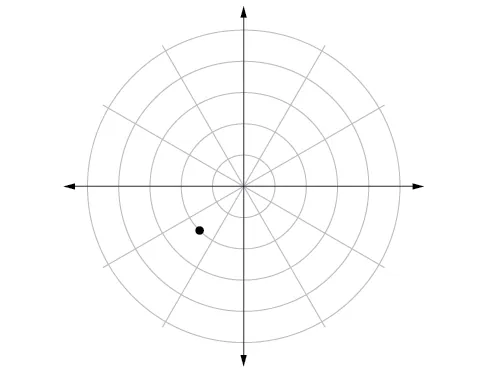55.

$r= 6 5cosθ−sinθ r= 6 5cosθ−sinθ$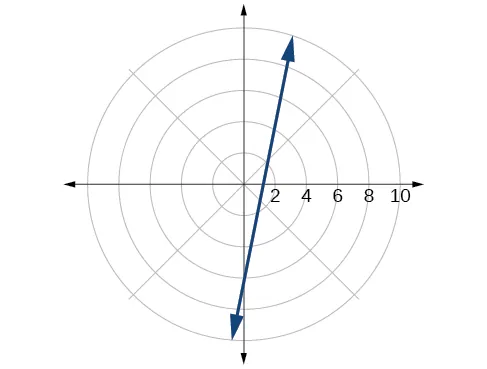57.

$r=2sinθ r=2sinθ$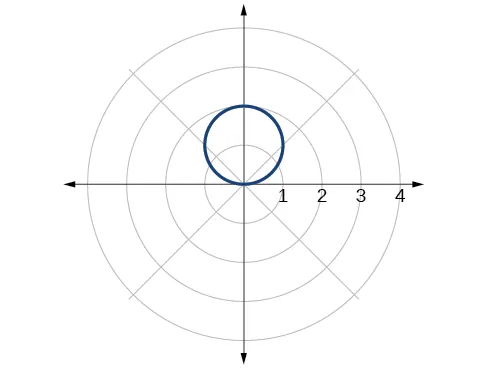59.

$r= 2 cosθ r= 2 cosθ$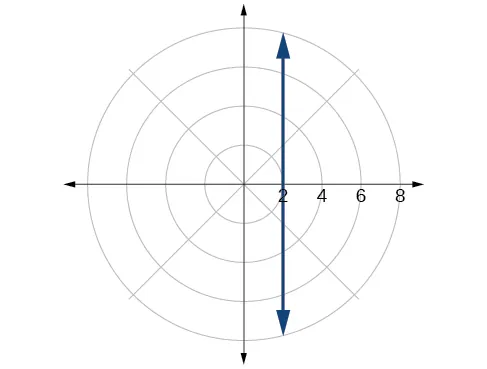61.

$r=3cosθ r=3cosθ$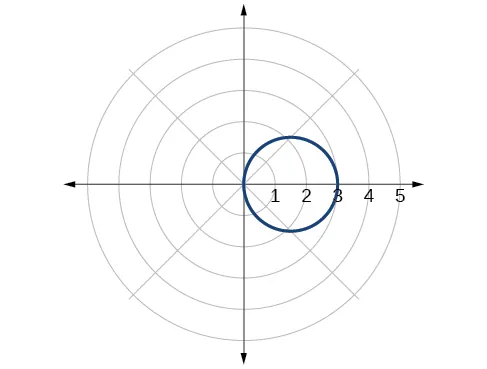63.

$x 2 + y 2 =16 x 2 + y 2 =16$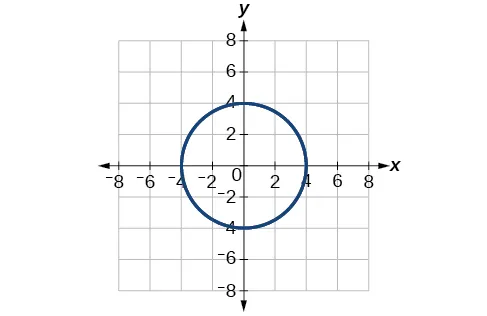65.

$y=x y=x$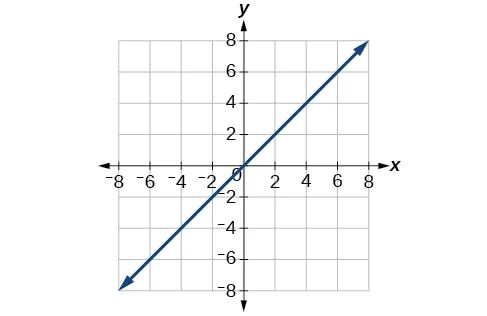67.

$x 2 + ( y+5 ) 2 =25 x 2 + ( y+5 ) 2 =25$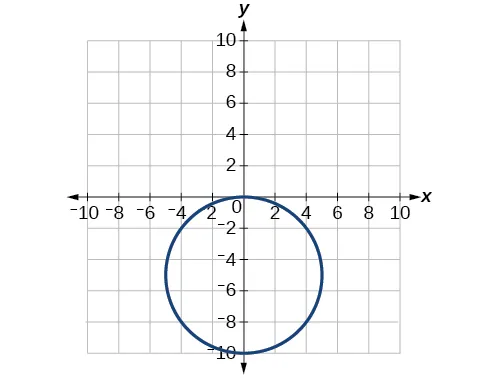69.

$( 1.618,−1.176 ) ( 1.618,−1.176 )$

71.

$( 10.630,131.186° ) ( 10.630,131.186° )$

73.

$( 2,3.14 )or( 2,π ) ( 2,3.14 )or( 2,π )$

75.

A vertical line with $a a$ units left of the y-axis.

77.

A horizontal line with $a a$ units below the x-axis.

79.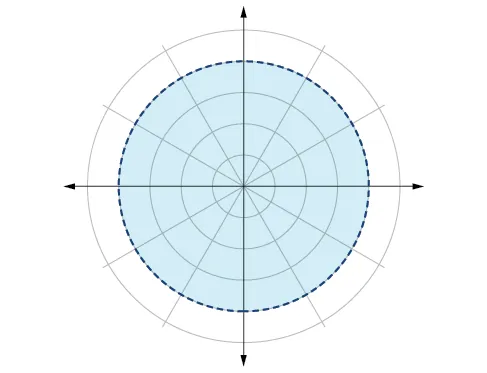81.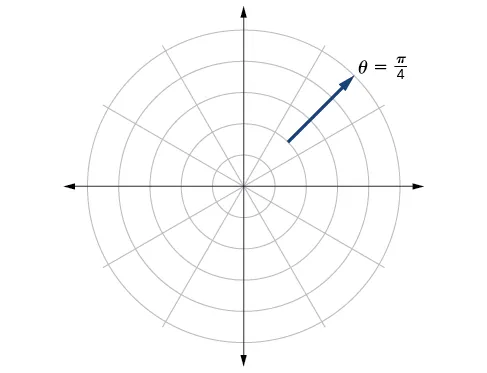83.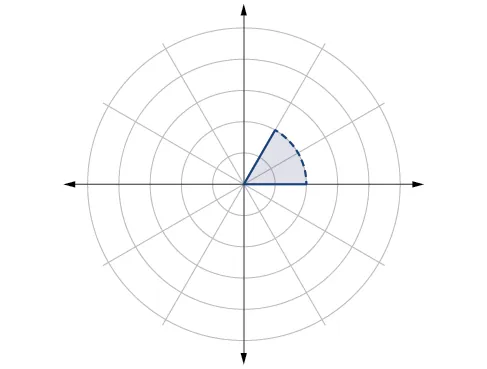### 8.4 Section Exercises

1.

Symmetry with respect to the polar axis is similar to symmetry about the $x x$-axis, symmetry with respect to the pole is similar to symmetry about the origin, and symmetric with respect to the line $θ= π 2 θ= π 2$ is similar to symmetry about the $y y$-axis.

3.

Test for symmetry; find zeros, intercepts, and maxima; make a table of values. Decide the general type of graph, cardioid, limaçon, lemniscate, etc., then plot points at $θ=0, π 2 , θ=0, π 2 ,$ $ππ$ and  $3π 2 , 3π 2 ,$ and sketch the graph.

5.

The shape of the polar graph is determined by whether or not it includes a sine, a cosine, and constants in the equation.

7.

symmetric with respect to the polar axis

9.

symmetric with respect to the polar axis, symmetric with respect to the line $θ= π 2 , θ= π 2 ,$ symmetric with respect to the pole

11.

no symmetry

13.

no symmetry

15.

symmetric with respect to the pole

17.

circle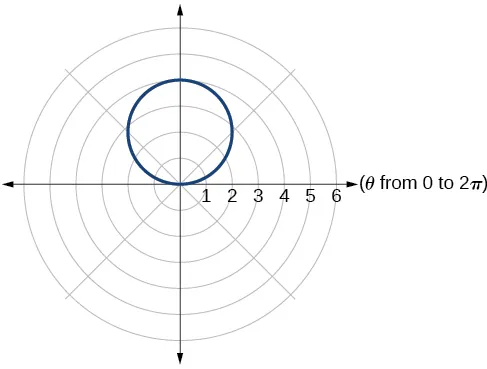19.

cardioid21.

cardioid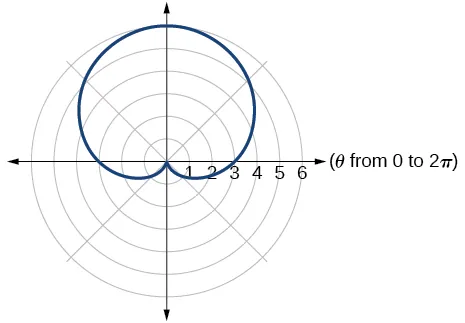23.

one-loop/dimpled limaçon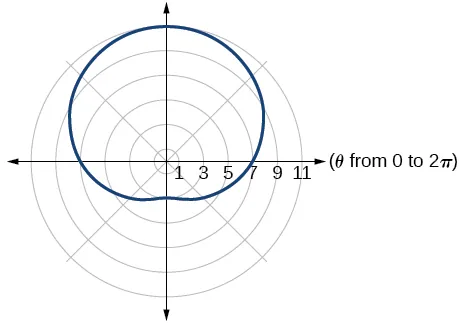25.

one-loop/dimpled limaçon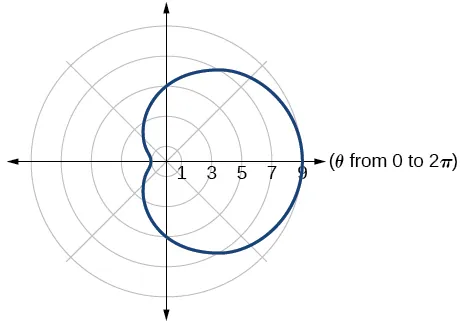27.

inner loop/two-loop limaçon29.

inner loop/two-loop limaçon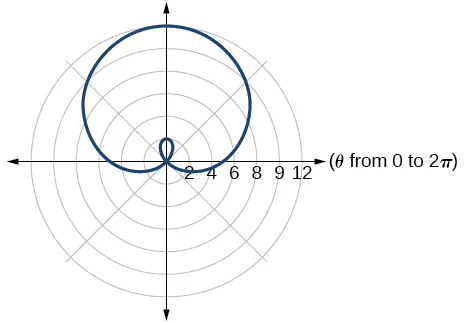31.

inner loop/two-loop limaçon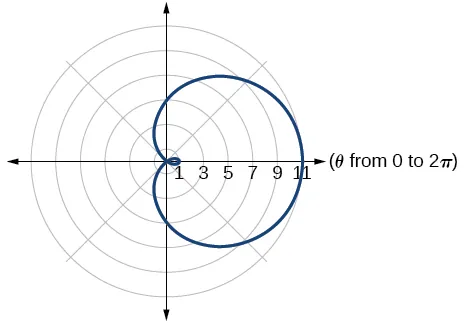33.

lemniscate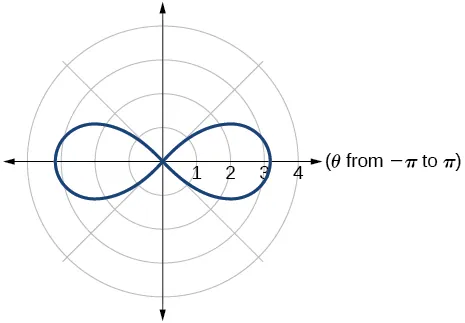35.

lemniscate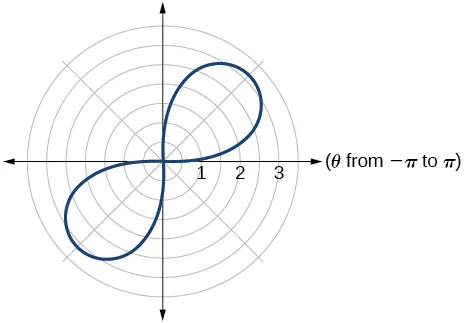37.

rose curve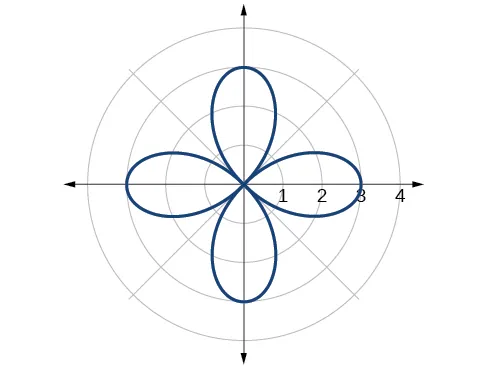39.

rose curve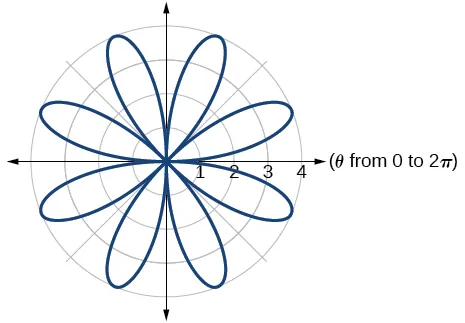41.

Archimedes’ spiral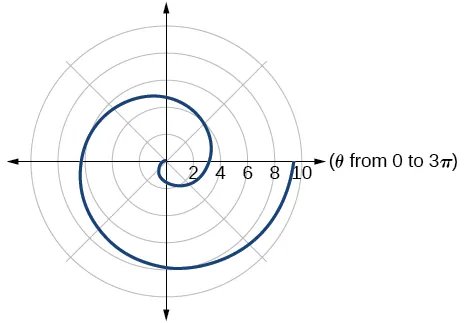43.

Archimedes’ spiral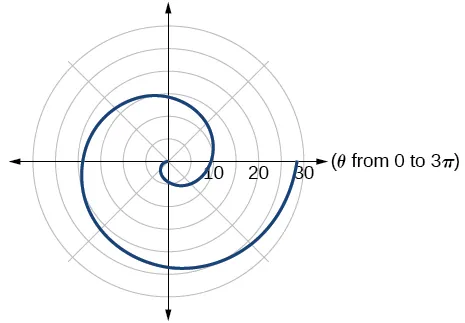45.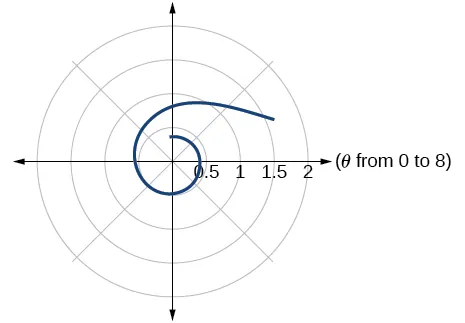47.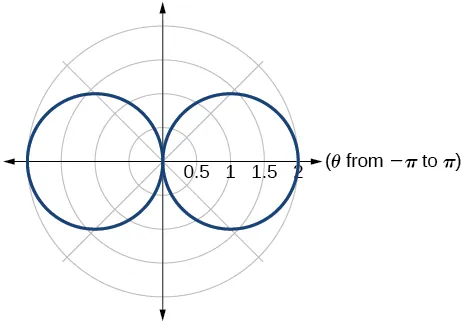49.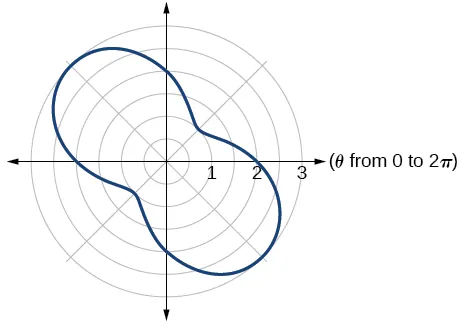51.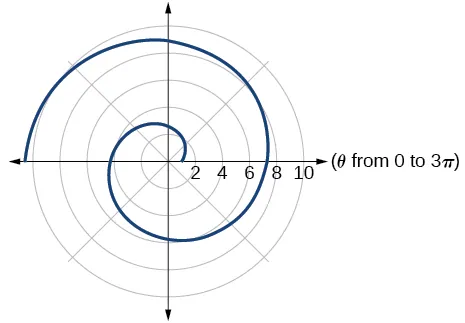53.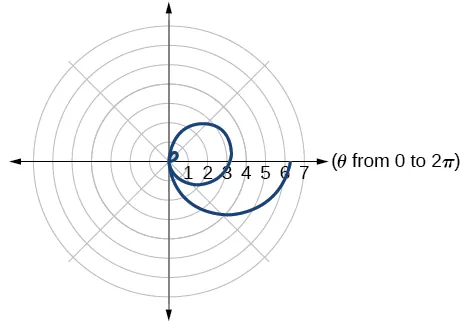55.

They are both spirals, but not quite the same.

57.

Both graphs are curves with 2 loops. The equation with a coefficient of $θ θ$ has two loops on the left, the equation with a coefficient of 2 has two loops side by side. Graph these from 0 to $4π 4π$ to get a better picture.

59.

When the width of the domain is increased, more petals of the flower are visible.

61.

The graphs are three-petal, rose curves. The larger the coefficient, the greater the curve’s distance from the pole.

63.

The graphs are spirals. The smaller the coefficient, the tighter the spiral.

65.

$( 4, π 3 ),( 4, 5π 3 ) ( 4, π 3 ),( 4, 5π 3 )$

67.

$( 3 2 , π 3 ),( 3 2 , 5π 3 ) ( 3 2 , π 3 ),( 3 2 , 5π 3 )$

69.

$( 0, π 2 ),( 0,π ),( 0, 3π 2 ),( 0,2π ) ( 0, π 2 ),( 0,π ),( 0, 3π 2 ),( 0,2π )$

71.

$( 8 4 2 , π 4 ),( 8 4 2 , 5π 4 ) ( 8 4 2 , π 4 ),( 8 4 2 , 5π 4 )$ and at $θ= 3π 4 , θ= 3π 4 ,$ $7π 4 7π 4$ since $r r$ is squared

### 8.5 Section Exercises

1.

a is the real part, b is the imaginary part, and $i= −1 i= −1$

3.

Polar form converts the real and imaginary part of the complex number in polar form using $x=rcosθ x=rcosθ$ and $y=rsinθ. y=rsinθ.$

5.

$z n = r n ( cos( nθ )+isin( nθ ) ) z n = r n ( cos( nθ )+isin( nθ ) )$ It is used to simplify polar form when a number has been raised to a power.

7.

$5 2 5 2$

9.

$38 38$

11.

$14.45 14.45$

13.

$4 5 cis( 333.4° ) 4 5 cis( 333.4° )$

15.

$2cis( π 6 ) 2cis( π 6 )$

17.

$7 3 2 +i 7 2 7 3 2 +i 7 2$

19.

$−2 3 −2i −2 3 −2i$

21.

$−1.5−i 3 3 2 −1.5−i 3 3 2$

23.

$4 3 cis( 198° ) 4 3 cis( 198° )$

25.

$3 4 cis( 180° ) 3 4 cis( 180° )$

27.

$5 3 cis( 17π 24 ) 5 3 cis( 17π 24 )$

29.

$7cis( 70° ) 7cis( 70° )$

31.

$5cis( 80° ) 5cis( 80° )$

33.

$5cis( π 3 ) 5cis( π 3 )$

35.

$125cis( 135° ) 125cis( 135° )$

37.

$9cis( 240° ) 9cis( 240° )$

39.

$cis( 3π 4 ) cis( 3π 4 )$

41.

$3cis( 80° ),3cis( 200° ),3cis( 320° ) 3cis( 80° ),3cis( 200° ),3cis( 320° )$

43.

$2 4 3 cis( 2π 9 ),2 4 3 cis( 8π 9 ),2 4 3 cis( 14π 9 ) 2 4 3 cis( 2π 9 ),2 4 3 cis( 8π 9 ),2 4 3 cis( 14π 9 )$

45.

$2 2 cis( 7π 8 ),2 2 cis( 15π 8 ) 2 2 cis( 7π 8 ),2 2 cis( 15π 8 )$

47.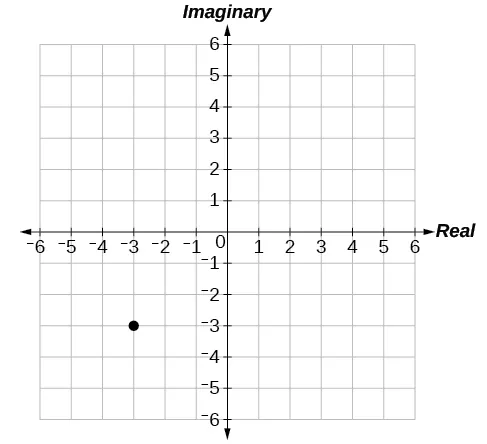49.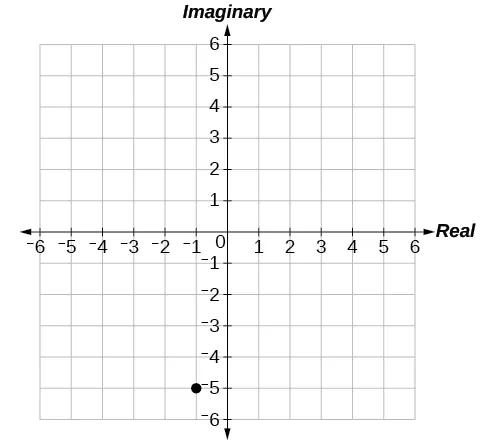51.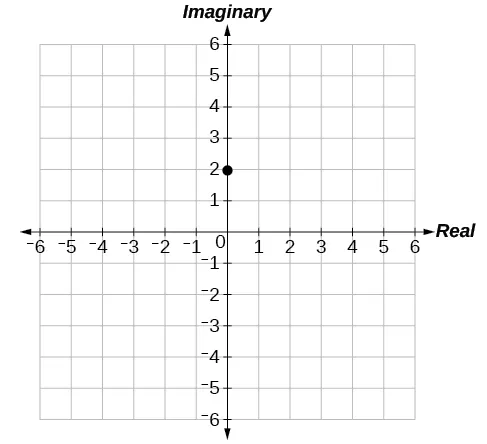53.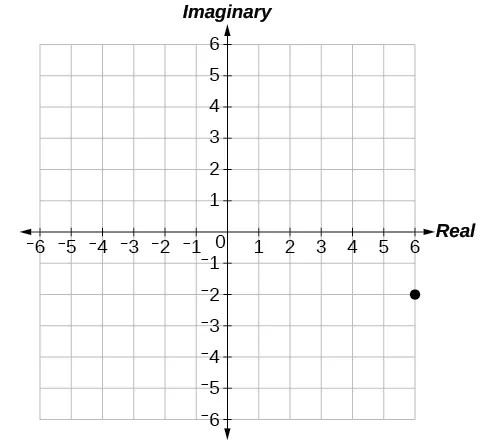55.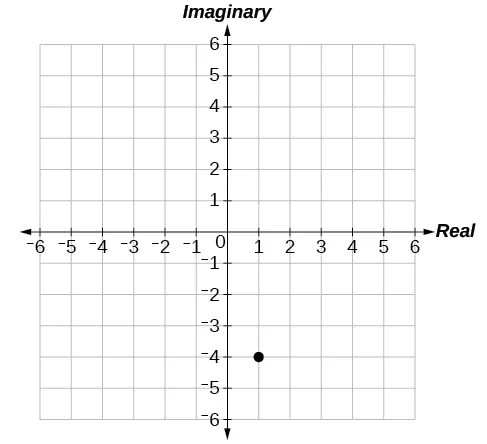57.

$3.61 e −0.59i 3.61 e −0.59i$

59.

$−2+3.46i −2+3.46i$

61.

$−4.33−2.50i −4.33−2.50i$

### 8.6 Section Exercises

1.

A pair of functions that is dependent on an external factor. The two functions are written in terms of the same parameter. For example, $x=f( t ) x=f( t )$ and $y=f( t ). y=f( t ).$

3.

Choose one equation to solve for $t, t,$ substitute into the other equation and simplify.

5.

Some equations cannot be written as functions, like a circle. However, when written as two parametric equations, separately the equations are functions.

7.

$y=−2+2x y=−2+2x$

9.

$y=3 x−1 2 y=3 x−1 2$

11.

$x=2 e 1−y 5 x=2 e 1−y 5$ or $y=1−5ln( x 2 ) y=1−5ln( x 2 )$

13.

$x=4log( y−3 2 ) x=4log( y−3 2 )$

15.

$x= ( y 2 ) 3 − y 2 x= ( y 2 ) 3 − y 2$

17.

$y= x 3 y= x 3$

19.

$( x 4 ) 2 + ( y 5 ) 2 =1 ( x 4 ) 2 + ( y 5 ) 2 =1$

21.

$y 2 =1− 1 2 x y 2 =1− 1 2 x$

23.

$y= x 2 +2x+1 y= x 2 +2x+1$

25.

$y= ( x+1 2 ) 3 −2 y= ( x+1 2 ) 3 −2$

27.

$y=−3x+14 y=−3x+14$

29.

$y=x+3 y=x+3$

31.

${ x( t )=t y( t )=2sint+1 { x( t )=t y( t )=2sint+1$

33.

${ x( t )= t +2t y( t )=t { x( t )= t +2t y( t )=t$

35.

${ x( t )=4cost y( t )=6sint ; { x( t )=4cost y( t )=6sint ;$ Ellipse

37.

${ x( t )= 10 cost y( t )= 10 sint ; { x( t )= 10 cost y( t )= 10 sint ;$ Circle

39.

${ x( t )=−1+4t y( t )=−2t { x( t )=−1+4t y( t )=−2t$

41.

${ x( t )=4+2t y( t )=1−3t { x( t )=4+2t y( t )=1−3t$

43.

yes, at $t=2 t=2$

45.
$t t$ $x x$ $y y$
1 -3 1
2 0 7
3 5 17
47.

49.

### 8.7 Section Exercises

1.

plotting points with the orientation arrow and a graphing calculator

3.

The arrows show the orientation, the direction of motion according to increasing values of $t. t.$

5.

The parametric equations show the different vertical and horizontal motions over time.

7.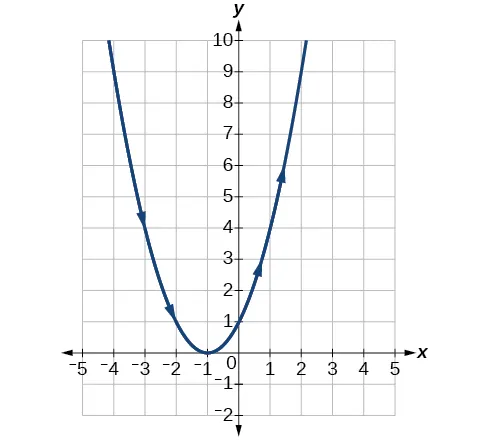9.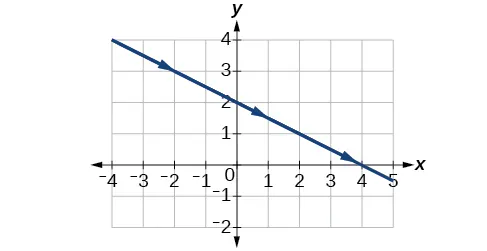11.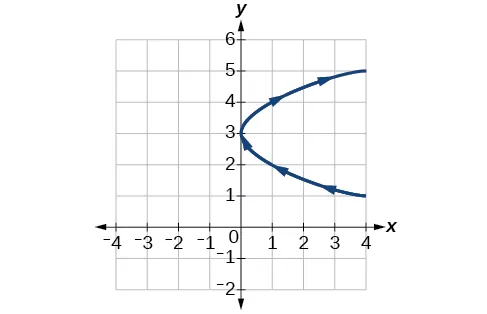13.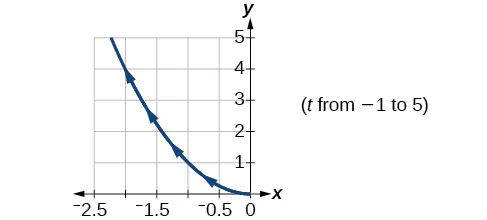15.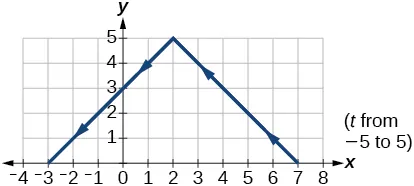17.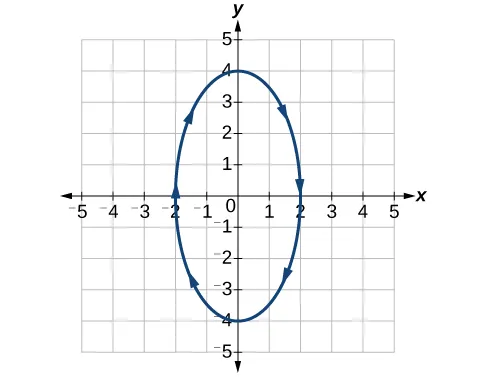19.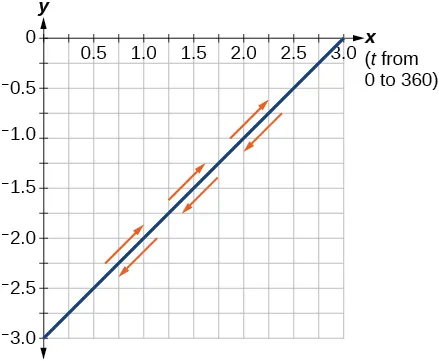21.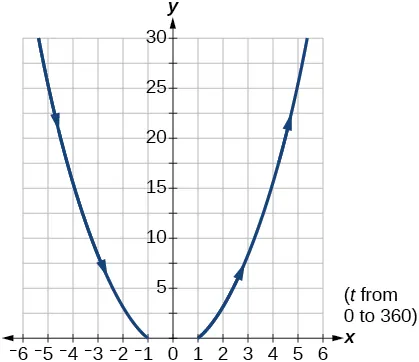23.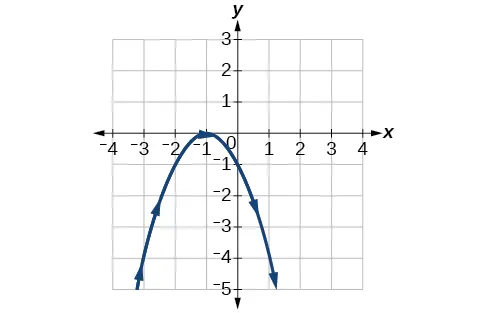25.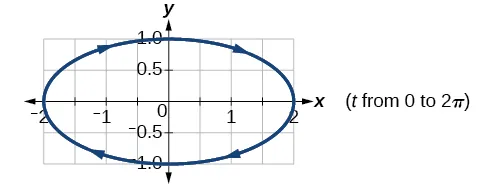27.29.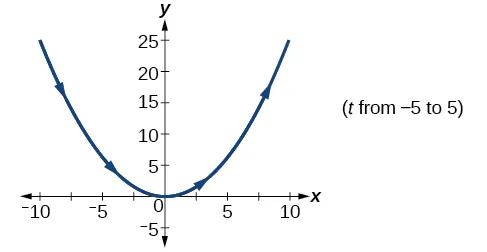31.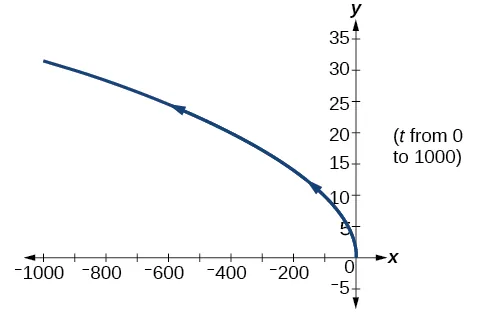33.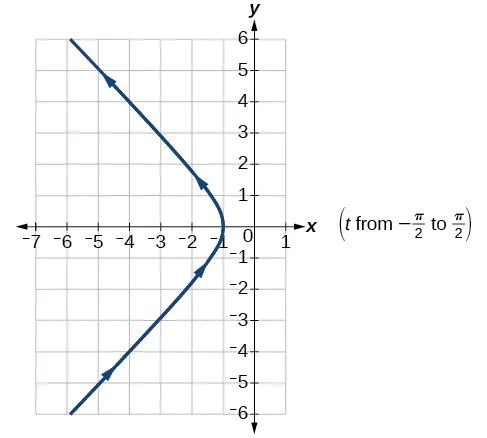35.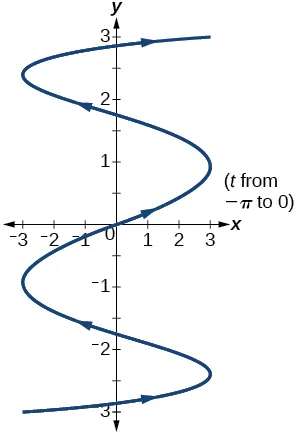37.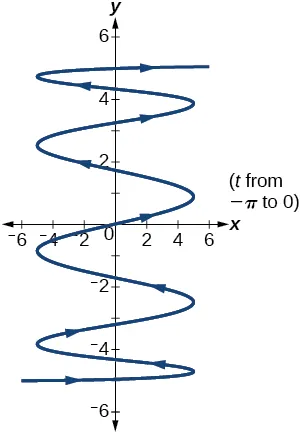39.

There will be 100 back-and-forth motions.

41.

Take the opposite of the $x( t ) x( t )$ equation.

43.

The parabola opens up.

45.

${ x( t )=5cost y( t )=5sint { x( t )=5cost y( t )=5sint$

47.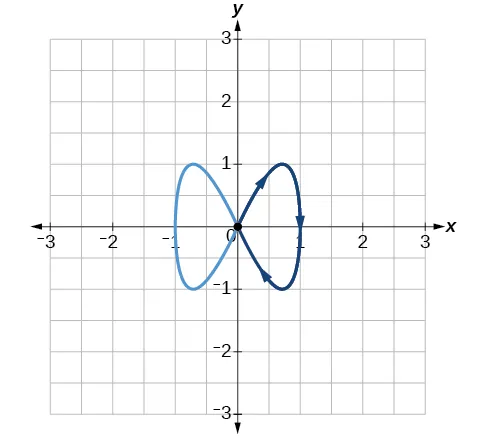49.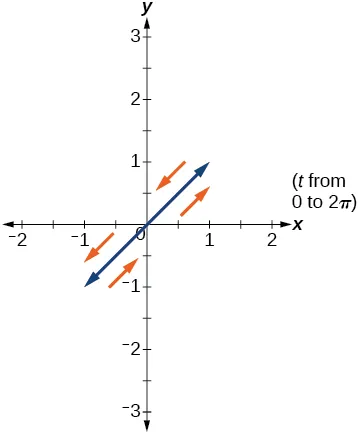51.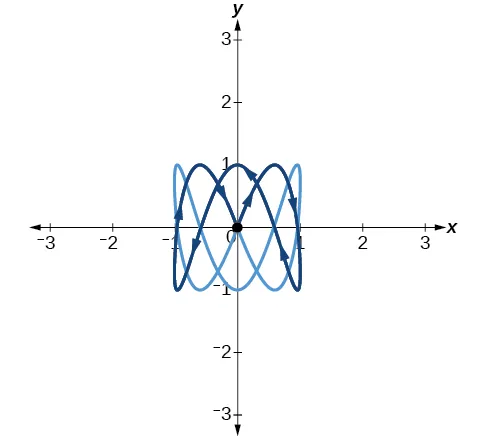53.

$a=4, a=4,$ $b=3,b=3,$ $c=6,c=6,$ $d=1 d=1$

55.

$a=4, a=4,$ $b=2,b=2,$ $c=3,c=3,$ $d=3 d=3$

57.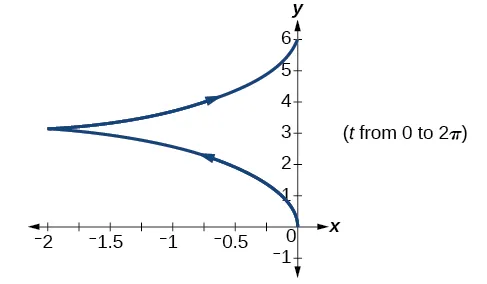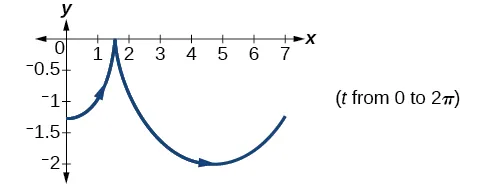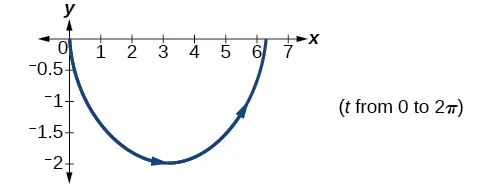59.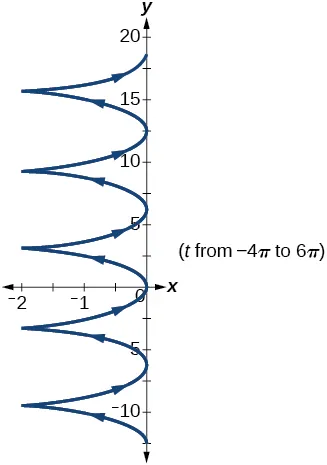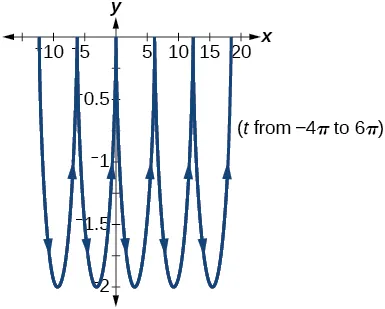61.

The $y y$-intercept changes.

63.

$y( x )=−16 ( x 15 ) 2 +20( x 15 ) y( x )=−16 ( x 15 ) 2 +20( x 15 )$

65.

${ x(t)=64tcos( 52° ) y(t)=−16 t 2 +64tsin( 52° ) { x(t)=64tcos( 52° ) y(t)=−16 t 2 +64tsin( 52° )$

67.

approximately 3.2 seconds

69.

1.6 seconds

71.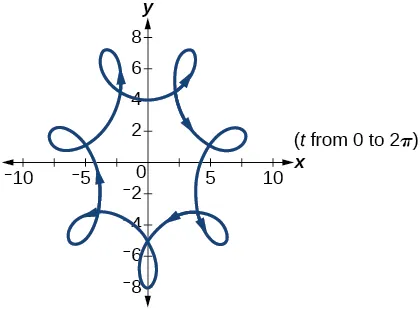73.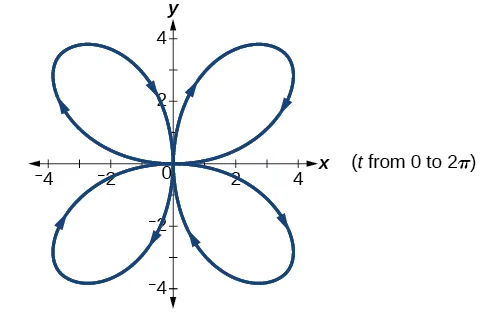### 8.8 Section Exercises

1.

lowercase, bold letter, usually $u,v,w u,v,w$

3.

They are unit vectors. They are used to represent the horizontal and vertical components of a vector. They each have a magnitude of 1.

5.

The first number always represents the coefficient of the $i, i,$ and the second represents the $j. j.$

7.

$〈 7,−5 〉 〈 7,−5 〉$

9.

not equal

11.

equal

13.

equal

15.

$7i−3j 7i−3j$

17.

$−6i−2j −6i−2j$

19.

$u+v=〈 −5,5 〉,u−v=〈 −1,3 〉,2u−3v=〈 0,5 〉 u+v=〈 −5,5 〉,u−v=〈 −1,3 〉,2u−3v=〈 0,5 〉$

21.

$−10i–4j −10i–4j$

23.

$− 2 29 29 i+ 5 29 29 j − 2 29 29 i+ 5 29 29 j$

25.

$− 2 229 229 i+ 15 229 229 j − 2 229 229 i+ 15 229 229 j$

27.

$− 7 2 10 i+ 2 10 j − 7 2 10 i+ 2 10 j$

29.

$| v |=7.810,θ=39.806° | v |=7.810,θ=39.806°$

31.

$| v |=7.211,θ=236.310° | v |=7.211,θ=236.310°$

33.

$−6 −6$

35.

$−12 −12$

37.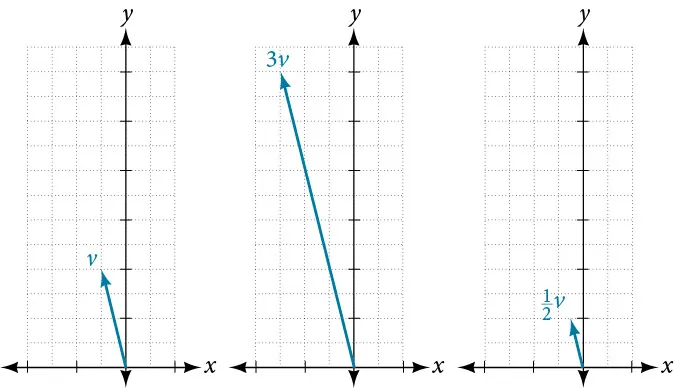39.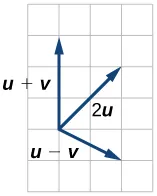41.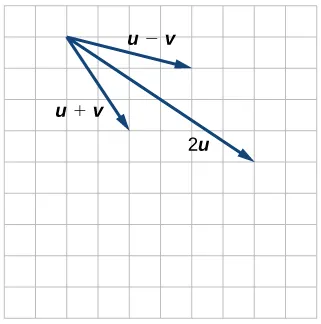43.45.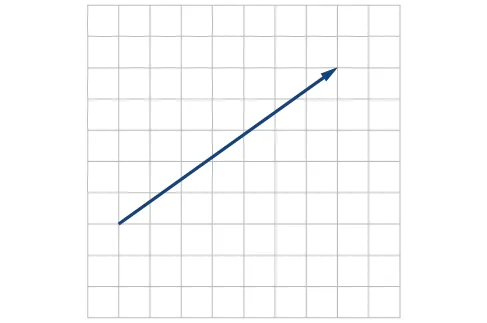47.

$〈 4,1 〉 〈 4,1 〉$

49.

$v=−7i+3j v=−7i+3j$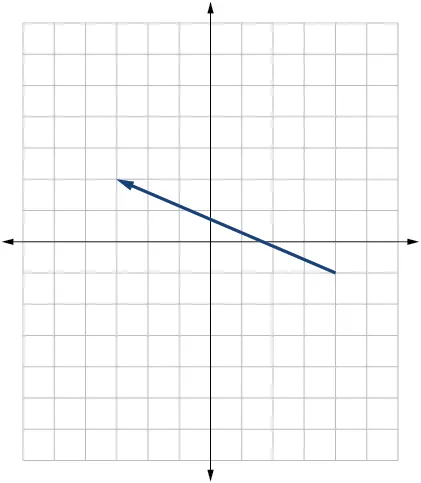51.

$3 2 i+3 2 j 3 2 i+3 2 j$

53.

$i− 3 j i− 3 j$

55.
1. 58.7
2. 12.5
57.

$x=7.13 x=7.13$ pounds, $y=3.63 y=3.63$ pounds

59.

$x=2.87 x=2.87$ pounds, $y=4.10 y=4.10$ pounds

61.

4.635 miles, 17.764° N of E

63.

17 miles. 10.318 miles

65.

Distance: 2.868. Direction: 86.474° North of West, or 3.526° West of North

67.

4.924°. 659 km/hr

69.

4.424°

71.

$( 0.081,8.602 ) ( 0.081,8.602 )$

73.

21.801°, relative to the car’s forward direction

75.

parallel: 16.28, perpendicular: 47.28 pounds

77.

19.35 pounds, 231.54° from the horizontal

79.

5.1583 pounds, 75.8° from the horizontal

### Review Exercises

1.

Not possible

3.

$C=120°,a=23.1,c=34.1 C=120°,a=23.1,c=34.1$

5.

distance of the plane from point $A: A:$ 2.2 km, elevation of the plane: 1.6 km

7.

$b=71.0°,C=55.0°,a=12.8 b=71.0°,C=55.0°,a=12.8$

9.

40.6 km

11.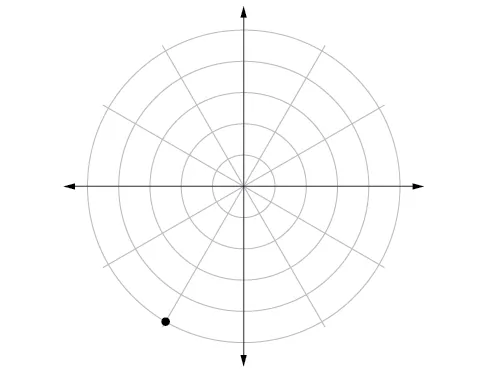13.

$( 0,2 ) ( 0,2 )$

15.

$( 9.8489,203.96° ) ( 9.8489,203.96° )$

17.

$r=8 r=8$

19.

$x 2 + y 2 =7x x 2 + y 2 =7x$

21.

$y=−x y=−x$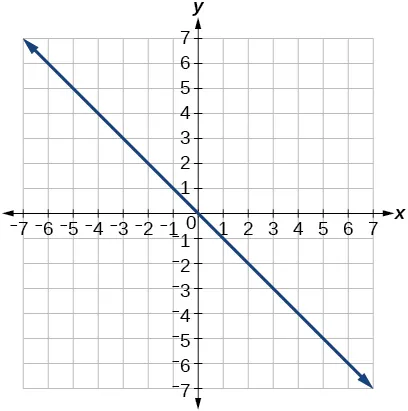23.

symmetric with respect to the line $θ= π 2 θ= π 2$

25.27.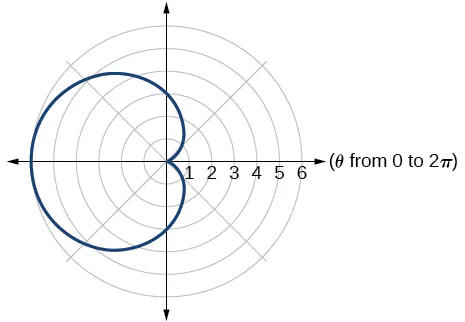29.

5

31.

$cis( − π 3 ) cis( − π 3 )$

33.

$2.3+1.9i 2.3+1.9i$

35.

$60cis( π 2 ) 60cis( π 2 )$

37.

$3cis( 4π 3 ) 3cis( 4π 3 )$

39.

$25cis( 3π 2 ) 25cis( 3π 2 )$

41.

$5cis( 3π 4 ),5cis( 7π 4 ) 5cis( 3π 4 ),5cis( 7π 4 )$

43.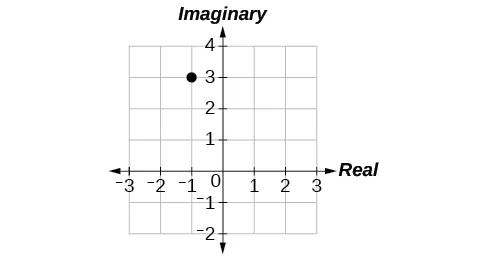45.

$x 2 + 1 2 y=1 x 2 + 1 2 y=1$

47.

${ x( t )=−2+6t y( t )=3+4t { x( t )=−2+6t y( t )=3+4t$

49.

$y=−2 x 5 y=−2 x 5$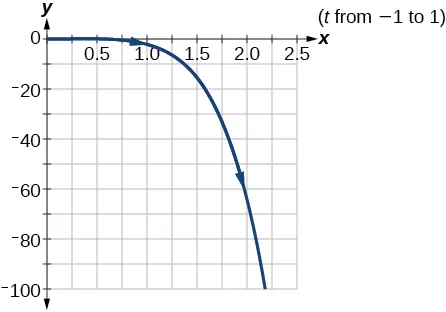51.
1. ${ x( t )=( 80cos( 40° ) )t y( t )=−16 t 2 +( 80sin( 40° ) )t+4 { x( t )=( 80cos( 40° ) )t y( t )=−16 t 2 +( 80sin( 40° ) )t+4$
2. The ball is 14 feet high and 184 feet from where it was launched.
3. 3.3 seconds
53.

not equal

55.

4i

57.

$− 3 10 10 − 3 10 10$ i $− 10 10 − 10 10$ j

59.

Magnitude: $3 2 , 3 2 ,$ Direction: $225° 225°$

61.

$16 16$

63.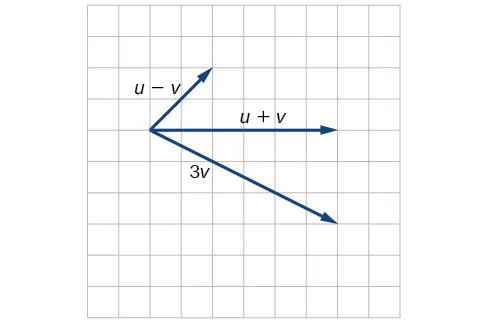### Practice Test

1.

$α=67.1°,γ=44.9°,a=20.9 α=67.1°,γ=44.9°,a=20.9$

3.

5.

$( 1, 3 ) ( 1, 3 )$

7.

$y=−3 y=−3$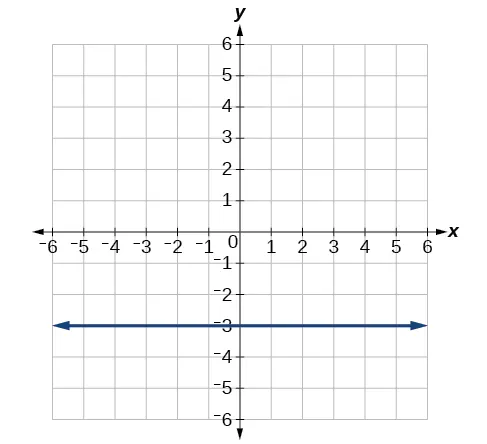9.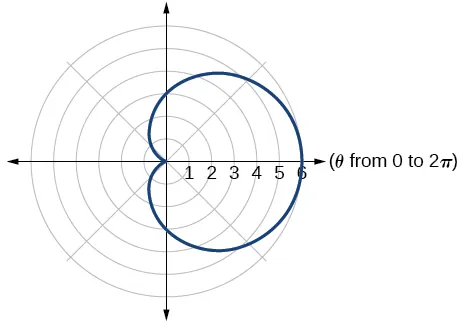11.

$106 106$

13.

$−5 2 +i 5 3 2 −5 2 +i 5 3 2$

15.

$4cis( 21° ) 4cis( 21° )$

17.

$2 2 cis( 18° ),2 2 cis( 198° ) 2 2 cis( 18° ),2 2 cis( 198° )$

19.

$y=2 ( x−1 ) 2 y=2 ( x−1 ) 2$

21.23.

−4i − 15j

25.

$2 13 13 i+ 3 13 13 j 2 13 13 i+ 3 13 13 j$

Order a print copy

As an Amazon Associate we earn from qualifying purchases.